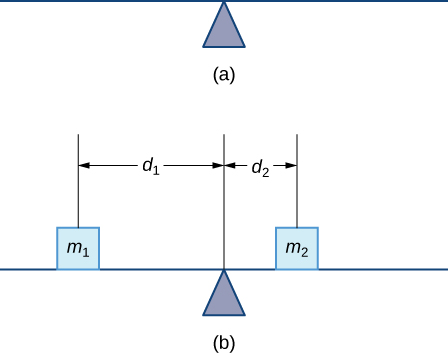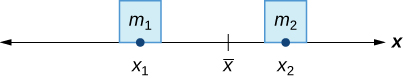# 6.6 Moments and centers of mass

 Page 1 / 14
• Find the center of mass of objects distributed along a line.
• Locate the center of mass of a thin plate.
• Use symmetry to help locate the centroid of a thin plate.
• Apply the theorem of Pappus for volume.

In this section, we consider centers of mass (also called centroids , under certain conditions) and moments. The basic idea of the center of mass is the notion of a balancing point. Many of us have seen performers who spin plates on the ends of sticks. The performers try to keep several of them spinning without allowing any of them to drop. If we look at a single plate (without spinning it), there is a sweet spot on the plate where it balances perfectly on the stick. If we put the stick anywhere other than that sweet spot, the plate does not balance and it falls to the ground. (That is why performers spin the plates; the spin helps keep the plates from falling even if the stick is not exactly in the right place.) Mathematically, that sweet spot is called the center of mass of the plate .

In this section, we first examine these concepts in a one-dimensional context, then expand our development to consider centers of mass of two-dimensional regions and symmetry. Last, we use centroids to find the volume of certain solids by applying the theorem of Pappus.

## Center of mass and moments

Let’s begin by looking at the center of mass in a one-dimensional context. Consider a long, thin wire or rod of negligible mass resting on a fulcrum, as shown in [link] (a). Now suppose we place objects having masses ${m}_{1}$ and ${m}_{2}$ at distances ${d}_{1}$ and ${d}_{2}$ from the fulcrum, respectively, as shown in [link] (b).(a) A thin rod rests on a fulcrum. (b) Masses are placed on the rod.

The most common real-life example of a system like this is a playground seesaw, or teeter-totter, with children of different weights sitting at different distances from the center. On a seesaw, if one child sits at each end, the heavier child sinks down and the lighter child is lifted into the air. If the heavier child slides in toward the center, though, the seesaw balances. Applying this concept to the masses on the rod, we note that the masses balance each other if and only if ${m}_{1}{d}_{1}={m}_{2}{d}_{2}.$

In the seesaw example, we balanced the system by moving the masses (children) with respect to the fulcrum. However, we are really interested in systems in which the masses are not allowed to move, and instead we balance the system by moving the fulcrum. Suppose we have two point masses, ${m}_{1}$ and ${m}_{2},$ located on a number line at points ${x}_{1}$ and ${x}_{2},$ respectively ( [link] ). The center of mass, $\stackrel{–}{x},$ is the point where the fulcrum should be placed to make the system balance.The center of mass x – is the balance point of the system.

Thus, we have

$\begin{array}{ccc}\hfill {m}_{1}|{x}_{1}-\stackrel{–}{x}|& =\hfill & {m}_{2}|{x}_{2}-\stackrel{–}{x}|\hfill \\ \hfill {m}_{1}\left(\stackrel{–}{x}-{x}_{1}\right)& =\hfill & {m}_{2}\left({x}_{2}-\stackrel{–}{x}\right)\hfill \\ \hfill {m}_{1}\stackrel{–}{x}-{m}_{1}{x}_{1}& =\hfill & {m}_{2}{x}_{2}-{m}_{2}\stackrel{–}{x}\hfill \\ \hfill \stackrel{–}{x}\left({m}_{1}+{m}_{2}\right)& =\hfill & {m}_{1}{x}_{1}+{m}_{2}{x}_{2}\hfill \\ \hfill \stackrel{–}{x}& =\hfill & \frac{{m}_{1}{x}_{1}+{m}_{2}{x}_{2}}{{m}_{1}+{m}_{2}}.\hfill \end{array}$

The expression in the numerator, ${m}_{1}{x}_{1}+{m}_{2}{x}_{2},$ is called the first moment of the system with respect to the origin. If the context is clear, we often drop the word first and just refer to this expression as the moment    of the system. The expression in the denominator, ${m}_{1}+{m}_{2},$ is the total mass of the system. Thus, the center of mass    of the system is the point at which the total mass of the system could be concentrated without changing the moment.

Given that u = tan–¹(y/x), show that d²u/dx² + d²u/dy²=0
find the limiting value of 5n-3÷2n-7
Use the first principal to solve the following questions 5x-1
175000/9*100-100+164294/9*100-100*4
mode of (x+4) is equal to 10..graph it how?
66
ram
6
ram
6
Cajab
what is domain in calculus
nelson
integrals of 1/6-6x-5x²
derivative of (-x^3+1)%x^2
(-x^5+x^2)/100
(-5x^4+2x)/100
oh sorry it's (-x^3+1)÷x^2
Misha
-5x^4+2x
sorry I didn't understan A with that symbol
find the derivative of the following y=4^e5x y=Cos^2 y=x^inx , x>0 y= 1+x^2/1-x^2 y=Sin ^2 3x + Cos^2 3x please guys I need answer and solutions
differentiate y=(3x-2)^2(2x^2+5) and simplify the result
Ga
72x³-72x²+106x-60
okhiria
y= (2x^2+5)(3x+9)^2
lemmor
solve for dy/dx of y= 8x^3+5x^2-x+5
192x^2+50x-1
Daniel
are you sure? my answer is 24x^2+10x-1 but I'm not sure about my answer .. what do you think?
Ga
24x²+10x-1
Ga
yes
ok ok hehe thanks nice dp ekko hahaha
Ga
hahaha 😂❤️❤️❤️ welcome bro ❤️
Ga
y= (2x^2+5)(3x+9)^2
lemmor
can i join?
Fernando
yes of course
Jug
can anyone teach me integral calculus?
Jug
it's just the opposite of differential calculus
yhin
of coursr
okhiria
but i think, it's more complicated than calculus 1
Jug
Hello can someone help me with calculus one...
Jainaba
find the derivative of y= (2x+3)raise to 2 sorry I didn't know how to put the raise correctly
8x+12
Dhruv
8x+3
okhiria
d the derivative of y= e raised to power x
okhiria
rates of change and tangents to curves
how can find differential Calculus
Kyaw
derivative of ^5√1+x
can you help with this f(×)=square roots 3-4
oscar
using first principle, find the derivative of y=cosx^3
Approximate root 4 without a calculator
Approximate root 4.02 without using a calculator
Tinkeu
2.03
temigbe
unit of energy
Safiyo
< or = or >4.00000001
Fufa
2.01
Cajab
+-2
PRATICK
find the integral of tan
sec
PRATICK
sec
PRATICK
tan
dipeshByBy Anindyo MukhopadhyayByBy